﻿ 浅谈图的割点和割边（Tarjan）

### 浅谈图的割点和割边（Tarjan）

快要NOIP了，想着复习一下图论，然后就发现不太会写割点和割边了，而且之前还没有写过博客，所以今天来填个坑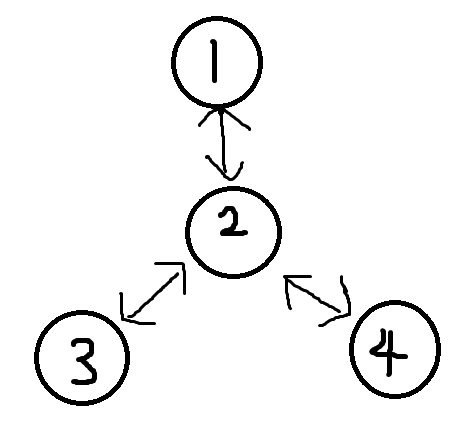1.割点可能有很多个

2.无向图和有向图都有割点

（1）当前点是根时，它的子树必须要超过两个，否则不是割点。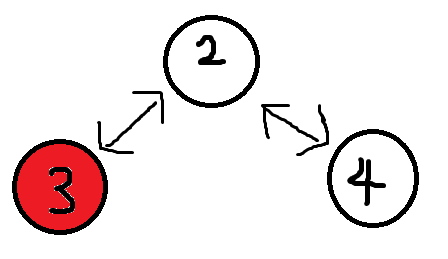（2）当前点不是根时，如果它有一个儿子在不经过它的情况下，不能够回到它的父亲，那么这个点就是割点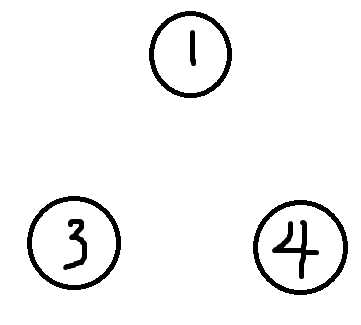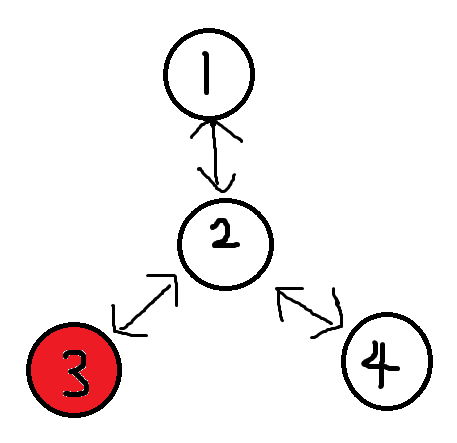LOW=1

DFN=2;

LOW=2;

DFN=3;

LOW=2;

DFN=0;

LOW=0;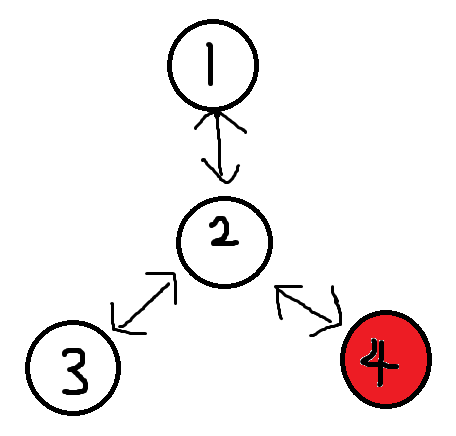LOW=1

DFN=2;

LOW=2;

DFN=3;

LOW=2;

DFN=4;

LOW=2;

4现在也没有儿子了，比较low和dfn，判定2为割点，回溯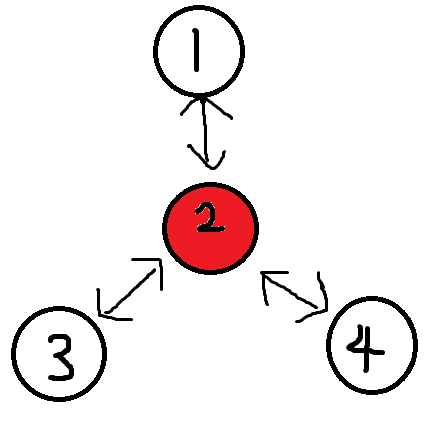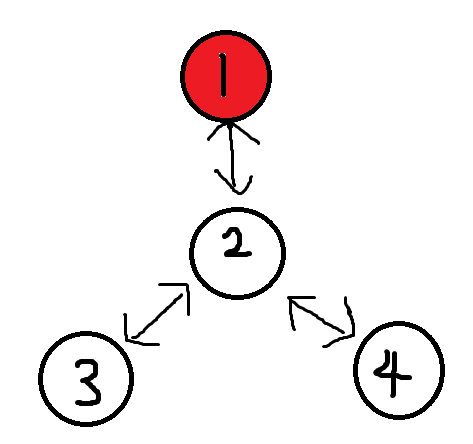4 3

1 2

2 3

2 4

2

```#include<iostream>
#include<cmath>
#include<cstdio>
#include<cstdlib>
#include<cstring>
#include<string>
#include<algorithm>
using namespace std;
inline int min(int a,int b){return a<b?a:b;}
inline int max(int a,int b){return a>b?a:b;}
inline int rd(){
int x=0,f=1;
char c=getchar();
for(;!isdigit(c);c=getchar()) if(c=='-') f=-1;
for(;isdigit(c);c=getchar()) x=x*10+c-'0';
return x*f;
}
inline void write(int x){
if(x<0) putchar('-'),x=-x;
if(x>9) write(x/10);
putchar(x%10+'0');
}
int n,m;
int nxt,to;
int total=0;
void add(int x,int y){
total++;
to[total]=y;
return ;
}
int dfn,low;
int tot=0;
int book;
void tarjan(int x,int fa){//记录一个fa，代表当前点的父亲
dfn[x]=low[x]=++tot;
int child=0;//以x为根的子树的个数
if(!dfn[to[e]]){
tarjan(to[e],x);//扩展
low[x]=min(low[x],low[to[e]]);//回溯low
if(x==fa) child++;//只有在当前点是根时用记录child
if(x!=fa&&low[to[e]]>=dfn[x]) book[x]=1;//如果一个点不是根，并且它的儿子在不经过它的情况下无法回到它的父亲，那么它也是割点
}
else if(to[e]!=fa) low[x]=min(low[x],dfn[to[e]]);//如果一个点被搜索过了并且是x的儿子或者是它的父亲，就可以直接回溯
}
if(x==fa&&child>=2) book[x]=1;//如果一个点是根并且有多于两个子树，就是割点
return ;
}
int main(){
n=rd(),m=rd();
for(int i=1;i<=m;i++){
int x=rd(),y=rd();
}
for(int i=1;i<=n;i++) if(!dfn[i]) tarjan(i,i);//防止图不连通
for(int i=1;i<=n;i++) if(book[i]) printf("%d ",i);//一个图可能有多个割点
return 0;
}```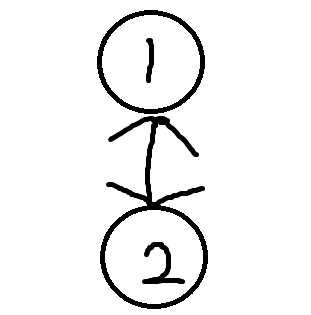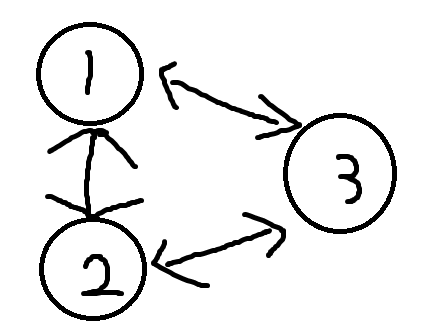2 1

1 2

1->2

```#include<iostream>
#include<cmath>
#include<cstdio>
#include<cstdlib>
#include<cstring>
#include<string>
#include<algorithm>
using namespace std;
inline int min(int a,int b){return a<b?a:b;}
inline int max(int a,int b){return a>b?a:b;}
inline int rd(){
int x=0,f=1;
char c=getchar();
for(;!isdigit(c);c=getchar()) if(c=='-') f=-1;
for(;isdigit(c);c=getchar()) x=x*10+c-'0';
return x*f;
}
inline void write(int x){
if(x<0) putchar('-'),x=-x;
if(x>9) write(x/10);
putchar(x%10+'0');
}
int n,m;
int nxt,to;
int total=0;
void add(int x,int y){
total++;
to[total]=y;
return ;
}
int dfn,low;
int tot=0;
void tarjan(int x,int fa){
dfn[x]=low[x]=++tot;
if(!dfn[to[e]]){
tarjan(to[e],x);//扩展
low[x]=min(low[x],low[to[e]]);//回溯low
if(low[to[e]]>dfn[x]) printf("%d->%d
",x,to[e]);//如果两点之间只有这一条路径，那么它是割边
}
else if(to[e]!=fa) low[x]=min(low[x],dfn[to[e]]);//如果一个点被搜索过了并且是x的儿子或者是它的父亲，就可以直接回溯
}
return ;
}
int main(){
n=rd(),m=rd();
for(int i=1;i<=m;i++){
int x=rd(),y=rd();